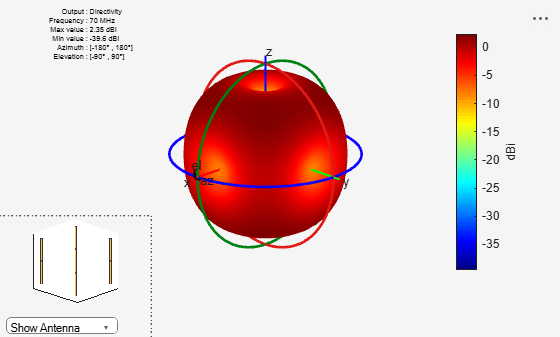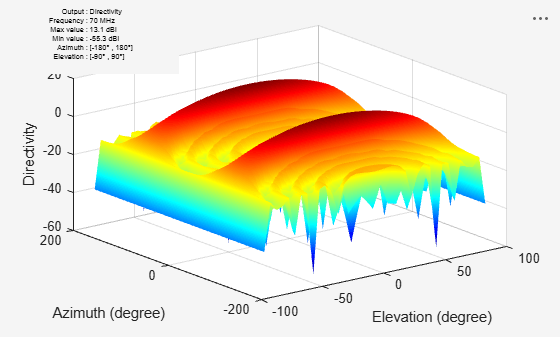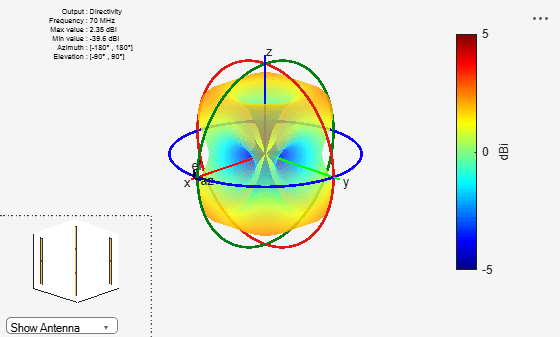# patternMultiply

Radiation pattern of array using pattern multiplication

## Syntax

``patternMultiply(array,frequency)``
``patternMultiply(array,frequency,azimuth)``
``patternMultiply(array,frequency,azimuth, elevation)``
``patternMultiply(___,Name,Value)``
``````[fieldval,azimuth,elevation] = patternMultiply(array,frequency)``````
``````[fieldval,azimuth,elevation] = patternMultiply(array,frequency,azimuth)``````
``````[fieldval,azimuth,elevation] = patternMultiply(array,frequency,azimuth,elevation)``````
``````[fieldval,azimuth,elevation] = patternMultiply(___,Name,Value)``````

## Description

example

````patternMultiply(array,frequency)` plots the 3-D radiation pattern of the array object over a specified frequency. `patternMultiply` calculates the full array pattern without taking the effect of mutual coupling between the different array elements.```
````patternMultiply(array,frequency,azimuth)` plots the radiation pattern of the array object for the given azimuth angles. Elevation angles retain default values.```
````patternMultiply(array,frequency,azimuth, elevation)` plots the radiation pattern of the array object for the given azimuth and elevation angles.```

example

````patternMultiply(___,Name,Value)` uses additional options specified by one or more `Name,Value` pair arguments. Specify name-value pair arguments after all other input arguments.```
``````[fieldval,azimuth,elevation] = patternMultiply(array,frequency)``` returns the field value such as the directivity of the lossless array in dBi or gain of the lossy array in dBi at the specified frequency. The size of the field value matrix is (number of elevation values) x (number of azimuth values). ```
``````[fieldval,azimuth,elevation] = patternMultiply(array,frequency,azimuth)``` returns the field value at the specified azimuth angles. Elevation angles retain default values.```
``````[fieldval,azimuth,elevation] = patternMultiply(array,frequency,azimuth,elevation)``` returns the field value at the specified azimuth angles, and elevation angles.```
``````[fieldval,azimuth,elevation] = patternMultiply(___,Name,Value)``` returns the field value using additional options specified by one or more `Name,Value` pair arguments. Specify name-value pair arguments after all other input arguments.```

## Examples

collapse all

Plot the radiation pattern of a default rectangular array at 70 MHz. Pattern multiplication does not take into consideration the effect of mutual coupling in array elements.

```h = rectangularArray; patternMultiply(h,70e6);```Plot the radiation pattern of a 10-element linear array at 70 MHz. Visualize the pattern using the rectangular coordinate system.

```l = linearArray('NumElements',10); patternMultiply(l,70e6,'CoordinateSystem','rectangular');```Plot the radiation pattern of a rectangular array with PatternOptions using a transparency of 0.6 and a magnitude scale of [-5 5].

```h = rectangularArray; p = PatternPlotOptions; p.Transparency = 0.6; p.MagnitudeScale = [-5 5]; patternMultiply(h, 70e6,'PatternOptions',p);```## Input Arguments

collapse all

Array object, specified as an object.

Example: `r = rectangularArray; patternMultiply(r,70e6)`. Plot the pattern of a rectangular array.

Frequency used to calculate array pattern, specified as a scalar in Hz.

Example: `70e6`

Data Types: `double`

Azimuth angle of the antenna, specified as a vector in degrees.

Example: `–90:5:90`

Data Types: `double`

Elevation angle of the antenna, specified as a vector in degrees.

Example: `0:1:360`

Data Types: `double`

### Name-Value Arguments

Example: `'CoordinateSystem'`, `rectangular`

Specify optional comma-separated pairs of `Name,Value` pair arguments. `Name` is the argument name and `Value` is the corresponding value. `Name` must appear inside single quotes (`''`). You can specify several name and value pair arguments in any order as ```Name1, Value1, ..., NameN, ValueN```.

Coordinate system of radiation pattern, specified as the comma-separated pair consisting of `'CoordinateSystem'` and one of these values: `'polar'`, `'rectangular'`, `'uv'`.

Example: `'CoordinateSystem'`, `'polar'`

Data Types: `char`

Value to plot, specified as a comma-separated pair consisting of `'Type'` and one of these values:

• `'directivity'` – Radiation intensity in a given direction of antenna in dB

• `'gain'` – Radiation intensity in a given direction of antenna, when the antenna has a lossy substrate in dB

• `'efield'` – Electric field of antenna in volt/meter

• `'power'` – Antenna power in watts

• `'powerdb'` – Antenna power in dB

Example: `'Type'`, `'efield'`

Data Types: `char`

Normalize field pattern, specified as the comma-separated pair consisting of `'Normalize'` and either `true` or `false`. For directivity patterns, this property is not applicable.

Example: `'Normalize'`, `false`

Data Types: `double`

Field polarization, specified as the comma-separated pair consisting of `'Polarization'` and one of these values:

• `'combined'`

• `'H'` – Horizontal polarization

• `'V'` – Vertical polarization

• `'RHCP'` – Right-hand circular polarization

• `'LHCP'` – Left-hand circular polarization

By default, you can visualize a combined polarization.

Example: `'Polarization'`, `'RHCP'`

Data Types: `char`

Parameter to change pattern plot properties, specified as the comma-separated pair consisting of `'PatternOptions'` and a `PatternPlotOptions` output. The properties that you can vary are:

• `Transparency`

• `SizeRatio`

• `AntennaOffset`

• `MagnitudeScale`

Example: `p = PatternPlotOptions('Transparency',0.1);` Create a pattern plot option with a transparency of 0.1. ```antennaarray = linearArray;````patternMultiply(antennaarray,75e6,'PatternOptions',p)`; Use this pattern plot option to visualize the pattern of a helix antenna.

Data Types: `double`

## Output Arguments

collapse all

Array directivity or gain, returned as a matrix in `dBi`. The matrix size is the product of the number of elevation values and azimuth values.

Azimuth angle used to calculate field values, returned as a vector in degrees.

Elevation angles used to calculate field values, returned as a vector in degrees.

## Version History

Introduced in R2017a